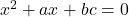1. Determine the nature of roots of each of the following equations
1.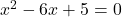2.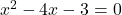3.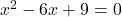4.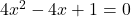5.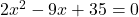6.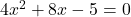2. For what values of p will the equation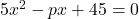?

3. if the equation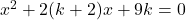has equal roots, find k.

4. For what value of a will the equation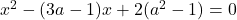have equal roots?

5. If the roots of the equation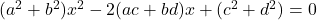are equal, then show that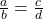6. Show that the roots of the equation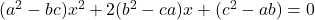will be equal, if either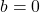or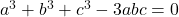7. If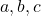are rational and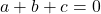, show that the roots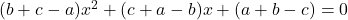are rational.

8. Prove that the roots of the equation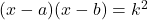are real for all values of k.

9. Show that the roots of the equation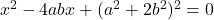are imaginary.

10. If the roots of the quadratic equation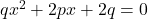are real and unequal, prove that the roots of the equation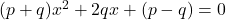are imaginary

1. From the equation whose roots are
1. 3,-2

2. -5,4

3.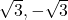4.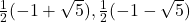5. -3+5i,-i-5i

6. a+ib,a-ib

1. Find a quadratic equation whose roots are twice the roots of2. Find a quadratic equation whose roots are reciprocals of the roots of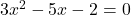3. Find a quadratic equation whose roots are greater by h than the roots of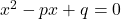4. Find a quadratic equation whose roots are the squares of the roots of2. Find a quadratic equation with rational coefficients one of whose roots is
1. 4+3i

2.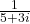3.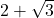3. Find the value of k so that the equation
1.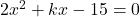has one root 3

2.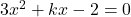has roots whose sum is equal to 6

3.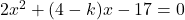has roots equal but opposite in sign

4.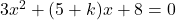has roots numerically equal but opposite in sign

5.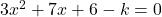has one root equal to zero

6.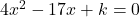has the reciprocal roots

7.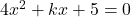has roots whose difference is4. Show that -1 is a root of the equation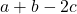x^2+(2a-b-c)x+(c+a-2b)=0\). Find the other root.

5. Find the value of m for which the equation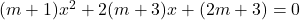will have (a) reciprocal roots (b) one root zero.

6. If the roots of the equation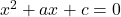differ by 1, prove that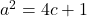7. If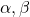are the roots of the equation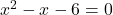, find the equation whose roots are
1.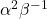and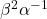2.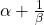and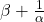8. Ifare the roots of the equation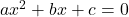, find the equation whose roots are
1.and2.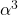and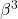3.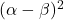and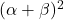4. the reciprocal of the roots of given equation

1. If the roots of the equationbe in the ratio of 3:4, prove that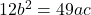2. If one root of the equationbe four times the other root, show that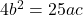3. For what values of m, the equation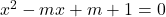may have its root in the ratio 2:3

1. Ifare the roots of the equation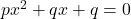, prove that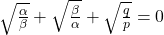2. If roots of the equation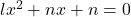be in the ratio of p:q, prove that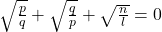9. If one root of the equationbe square of the other root, prove that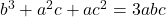1. Show that each pair of following equations has a common root
1.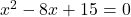and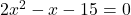2.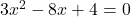and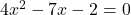2. Find the value of p so that each pair of the equations may have one root common
1.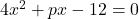and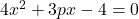2.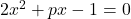and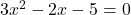3. If the quadratic equations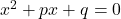and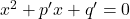have common roots show that it must be either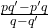or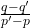4. If the quadratic equationsand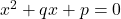have common roots show that it must be eitheror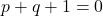5. If the quadratic equationsand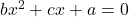have common roots show that it must be either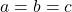or6. Prove that if the equations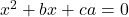and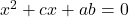have a common root, their other root will satisfy